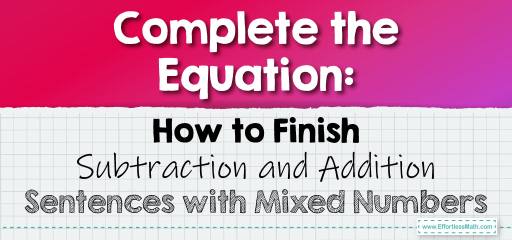# Complete the Equation: How to Finish Subtraction and Addition Sentences with Mixed Numbers

Mixed numbers, which combine whole numbers and fractions, are frequently encountered in various mathematical and real-world contexts.When presented with incomplete addition or subtraction sentences involving mixed numbers, the challenge lies in determining the missing value. In this guide, we’ll explore how to finish such sentences, ensuring a clear understanding of the operations involved.

## Step-by-step Guide to Complete the Equation:

1. Understanding the Sentence Structure:

Incomplete sentences will typically have a missing value, represented by a blank or a variable (like $$x$$). Your task is to determine this missing value.

– Begin by adding/subtracting the fractional parts. If the fractions have different denominators, find a common denominator.

– Next, add/subtract the whole numbers.

– Combine the results to get the final mixed number.

3. Approaching Subtraction Sentences:

For subtraction:

– Start by subtracting the fractional parts. If the fraction from which you are subtracting is larger than the first fraction, borrow from the whole number.

– Then, subtract the whole numbers.

– Combine the results to get the final mixed number.

Complete the sentence: $$2 \frac{1}{4} + 3 \frac{3}{4} = \_\_\_\_$$

Solution:

Adding the fractions: $$\frac{1}{4} + \frac{3}{4} = \frac{4}{4} = 1$$

Adding the whole numbers: $$2 + 3 + 1 (from the fractions) = 6$$

The completed sentence is: $$2 \frac{1}{4} + 3 \frac{3}{4} = 6$$

The Absolute Best Book for 5th Grade Students

### Example 2 (Subtraction):

Complete the sentence: $$5 \frac{3}{5} – 2 \frac{2}{5} = \_\_\_\_$$

Solution:

Subtracting the fractions: $$\frac{3}{5} – \frac{2}{5} = \frac{1}{5}$$

Subtracting the whole numbers: $$5 – 2 = 3$$

The completed sentence is: $$5 \frac{3}{5} – 2 \frac{2}{5} = 3 \frac{1}{5}$$

### Practice Questions:

1. Complete the sentence: $$4 \frac{2}{7} + 3 \frac{5}{7} = \_\_\_\_$$

2. Complete the sentence: $$7 \frac{3}{8} – 5 \frac{1}{8} = \_\_\_\_$$

3. Complete the sentence: $$6 \frac{1}{6} + 2 \frac{5}{6} = \_\_\_\_$$

A Perfect Book for Grade 5 Math Word Problems!

1. $$8$$

2. $$2 \frac{2}{8}$$ or $$2 \frac{1}{4}$$

3. $$9$$

The Best Math Books for Elementary Students

### What people say about "Complete the Equation: How to Finish Subtraction and Addition Sentences with Mixed Numbers - Effortless Math: We Help Students Learn to LOVE Mathematics"?

No one replied yet.

X
51% OFF

Limited time only!

Save Over 51%

SAVE $15 It was$29.99 now it is \$14.99